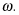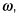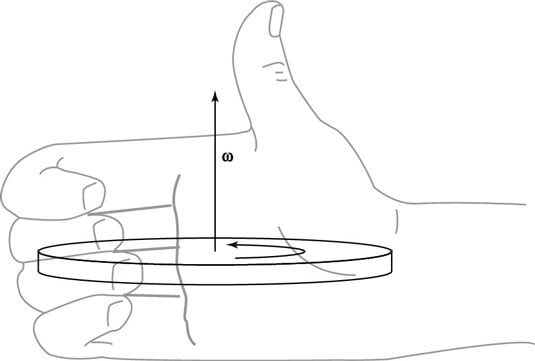##### Physics I For DummiesIn physics, when a wheel is spinning, it has not only an angular speed but also a direction. Here’s what the angular velocity vector tells you:

• The size of the angular velocity vector tells you the angular speed.

• The direction of the vector tells you the axis of the rotation, as well as whether the rotation is clockwise or counterclockwise.

Say that a wheel has a constant angular speed,Can you determine the direction in which its angular velocity,points? It can’t point along the rim of the wheel, as tangential velocity does, because its direction would then change every second. In fact, the only real choice for its direction is perpendicular to the wheel.

The direction of the angular velocity always takes people by surprise: Angular velocity,points along the axle of a wheel (as you can see in the figure).Angular velocity points in a direction perpendicular to the wheel.

Because the angular velocity vector points the way it does, it has no component along the wheel. The wheel is spinning, so the tangential (linear) velocity at any point on the wheel is constantly changing direction — except for at the very center point of the wheel, where the base of the angular velocity vector sits. If the wheel is lying flat on the ground, the vector’s head points up or down, away from the wheel, depending on which direction the wheel is rotating.

You can use the right-hand rule to determine the direction of the angular velocity vector. Wrap your right hand around the wheel so that your fingers point in the direction of the tangential motion at any point — the fingers on your right hand should go in the same direction as the wheel’s rotation. When you wrap your right hand around the wheel, your thumb points in the direction of the angular velocity vector,The figure shows a wheel lying flat, turning counterclockwise when viewed from above. Wrap your fingers in the direction of rotation. Your thumb, which represents the angular velocity vector, points up; it runs along the wheel’s axle. If the wheel were to turn clockwise instead, your thumb — and the vector — would have to point down, in the opposite direction.